# Civil Engineering - UPSC Civil Service Exam Questions

21.

If s is the shear strength, c and Φ are shear strength parameters, and σn is the normal stress at failure, then Coulomb's equation for shear strength of the soil can be represented by

 A. c = s + σs tan Φ B. c = s - σn tan Φ C. s = σn + c tan Φ D. s = c - σn tan Φ

Explanation:

No answer description available for this question. Let us discuss.

22.

The beam shown in the figure given above is subjected to concentrated load and clockwise couple. What is the vertical reaction at A ?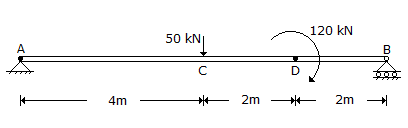A. 10 kN B. 40 kN C. 50 kN D. 30 kN

Explanation:

No answer description available for this question. Let us discuss.

23.

What is the vertical displacement at the point C of the structure shown in the figure ?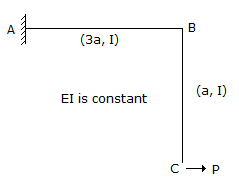A.B.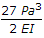C.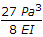D.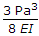Explanation:

No answer description available for this question. Let us discuss.

24.

If all the dimensions of a prismatic bar of square cross-section suspended freely from the ceiling of a roof are doubled then the total elongation produced by its own weight will increase

 A. eight times B. four times C. three times D. two times

Explanation:

No answer description available for this question. Let us discuss.

25.

Which of the following statements are true for the pile shown in the figure ?
1. Frictional resistance acts upwards through the length of the pile.
2. Negative skin friction acts over the length AB
3. Frictional resistance acts upwards over the length BC
4. There is point resistance at level C
Select the correct answer using the codes given below :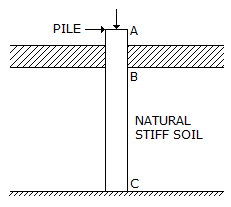A. 1, 3 and 4 B. 2, 3 and 4 C. 1 and 2 D. 2 and 3